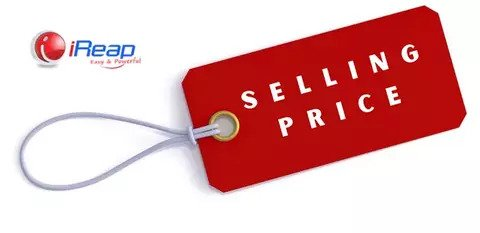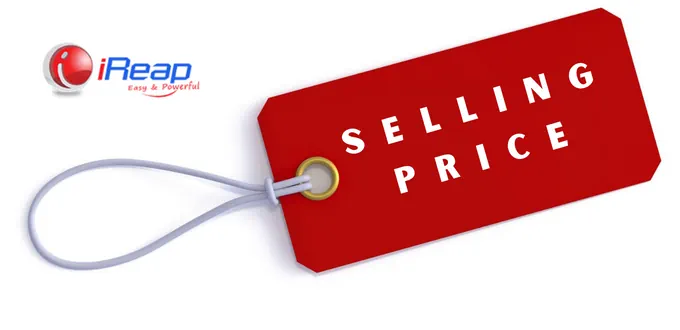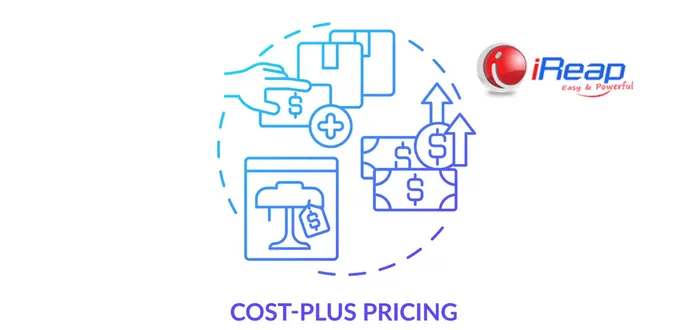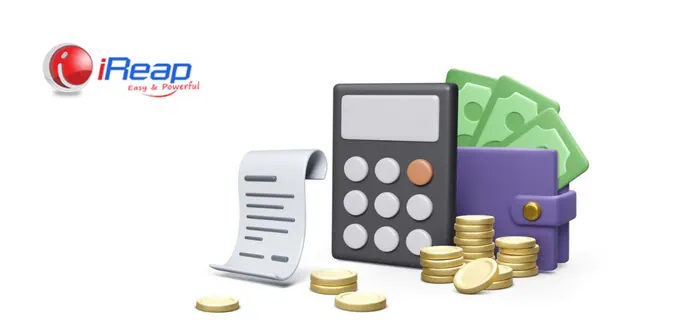# How to Determine and Calculate The Selling Price and its FormulaThe selling price results from the sum of costs, such as production costs, non-production costs, and the desired profit, which is then charged to consumers who wish to have products/services at that price. So, how do you determine and calculate the selling price? Read the full explanation below.

## Difference of Cost and Selling Price

Many sellers still think that the Cost of Goods Sold (HPP) is the same as the selling price. The introductory price is different from the selling price.

Cost of Goods Sold (HPP) is the number of expenses and costs, directly or indirectly, incurred by the company to produce goods or services. You can read an explanation about HPP here: How to Easily Determine Cost of Goods Sold (HPP) and Selling Price.

While the selling price is the price charged to consumers to obtain goods or services. Usually, the selling price includes the profit or profit that the seller wants.

## How to Determine the Selling Price

### 1. Determining Selling Price Based on Market Price

For products that have many competitors, you can set prices based on market prices or market-based pricing. Market prices are formed automatically in the market based on supply and demand. So, if you determine the selling price based on the market price, follow the general price circulating in the market without the hassle of picking and calculating the selling price again.

### 2. How to Determine Selling Price Based on Cost

Determining the selling price based on cost is known as breakeven pricing. Usually, this method is used by businesses that are just starting up and have yet to focus on making a profit. They are still introducing their products and only hope they can be sold; at least they can return the production costs first.### 3. Cost-Plus Pricing

Determining the selling price can use cost-plus pricing. To do this, you need to add the total production costs per number of products, then add the profit margin as you wish. If your product is homemade, you can use the cost-plus pricing method to determine the selling price of the product.

### 4. Markup Selling Price or Markup Pricing

Markup pricing is a method that increases the product price first to determine the selling price, then calculates the profit margin. This method is the opposite of cost-plus pricing, and you can use it to determine the selling price of products, both self-produced and products are taken from distributors.

### 5. Determining the Selling Price Using the Keystone Method

The Keystone Method is a method of determining the selling price of a product using an initial markup of 50% – 100% of the costs incurred (production costs, transportation costs, purchasing costs, and so on). This method is one of the retail businesses’ most widely used methods of determining prices because it makes it easier to apply discount strategies or other types of promotions.

### 6. Using the Highest Retail Price (HET)

The easiest way to determine the selling price is to use the Manufacturer Suggested Retail Price (MSRP), where you need to use the Highest Retail Price (HET) set by business associations in the same industry.

### 7. Determining the Selling Price of the Profit, You Want to Get

If you buy a product from a distributor for IDR 5,000, – and you want to get a profit of IDR 2,000 / product, then you can immediately set the selling price for IDR 7,000. Your selling price needs to be lowered and make the product not sell.## How to calculate selling price

### 1. How to Calculate Selling Prices Using the Breakeven Pricing Method

Because breakeven pricing does not focus on profits and only takes into account costs so that you can get a return on investment immediately, you need to use the breakeven pricing formula, namely:

Selling Price = (Fixed Cost + Variable Cost)/Number of Products.

Example:

You produce 100 pandan cakes daily with a fixed cost of Rp. Two million for purchasing materials and a variable cost of Rp. 3,000 per product. Then the calculation:

= IDR 2,000,000 + (IDR 3,000 x 100 loaves)/100
= (IDR 2,000,000 + IDR 300,000)/100
= IDR 2,300,000, – / 100
= IDR 23,000

So, the selling price of your product to get a quick return on investment is IDR 23,000 / pandan cake.

### 2. How to Calculate Selling Prices Using the Keystone Method

There is no specific formula to calculate the selling price using the keystone method. In principle, you only need to increase the product’s price using an initial markup of between 50% and 100% of the costs you incur to get the product.

Example:

You just bought wholesale work clothes for IDR 50,000/product. You immediately increase the price based on the initial markup above so that the selling price of work clothes is between 100 thousand – 150 thousand per product.

### 3. How to Calculate Selling Price Using the Cost-Plus Pricing Formula

For those of you who produce your products, then sell your products too, for example, a pastry business. You can use cost-plus pricing to determine the selling price of your pastries. Just use this cost-plus pricing formula:

Selling Price = [(Fixed Cost + Variable Cost)/Number of Products] + [Profit Margin X (Fixed Cost + Variable Cost)/Number of Products]

Example:

You can produce as many as 50 jars of pastries in one day. Raw material costs IDR 2,000,000, – daily electricity costs 200 thousand, labor costs IDR 300 thousand per day, and daily marketing costs IDR 100,000 – If your desired profit margin is 20% for each product, here’s how to calculate the price of selling pastries:

Total Cost: IDR 2,600,000,-
Profit margin: 20% x IDR 2,600,000 = IDR 520,000
Total Price: IDR 2,600,000 + IDR 520,000 = IDR 3,120,000

Then the selling price: IDR 62,400/product

For calculating the selling price of other products, you can also read from iReap’s article entitled How to Calculate Product Selling Prices to Get Big Profits.